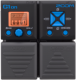# Engl Powerball Amp

Discussion in 'Zoom G1on/G1Xon' started by vap0r, May 22, 2021.

1. Engl Powerball AmpDevice: Zoom G1on
Firmware: 1.21

Name on device: POWERBALL
Optimized for: Phones/Speaker

Effects chain:This patch simulates a Engl Powerball Amplifier

Effect: "ZNR" (Dynamics / Filter), active - "yes"
"THRSH" = 13
"DETCT" = GtrIn
"Level" = 100

Effect: "ALIEN" (Amp simulators), active - "yes"
"Gain" = 77
"Tube" = 89
"Level" = 39
"Trebl" = 70
"Middl" = 51
"Bass" = 72
"Prese" = 82
"CAB" = ALIEN 4x12
"OUT" = LINE

Effect: "GraphicEQ" (Dynamics / Filter), active - "yes"
"160Hz" = 0
"400Hz" = 0
"800Hz" = 2
"3.2kHz" = 3
"6.4kHz" = 0
"12kHz" = 0
"Level" = 100

Effect: "ParaEQ" (Dynamics / Filter), active - "yes"
"Freq1" = 4.0kHz
"Q1" = 8
"Gain1" = -11
"Freq2" = 5.0kHz
"Q2" = 0.5
"Gain2" = 3
"Level" = 126

Effect: "ParaEQ" (Dynamics / Filter), active - "yes"
"Freq1" = 800Hz
"Q1" = 4
"Gain1" = -5
"Freq2" = 630Hz
"Q2" = 1
"Gain2" = 0
"Level" = 100

Patch Volume: 82

Note: This is a patch file, you will need to download and install the ToneLib-Zoom software to use the patch.

File size:
417 bytes
Views:
114